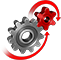# WolframSystemModeler

## Lotka–Volterra

The classic Lotka–Volterra model was originally proposed to explain variations in fish populations in the Mediterranean, but it has since been used to explain the dynamics of any predator-prey system in which certain assumptions are valid. Here, using SystemModeler, the oscillations of the snowshoe hare and the lynx are explored.

To run this example, you'll need

### The Lotka–Volterra Model as a Component-Based ModelThe Lotka–Volterra model in an intuitive component style created in Wolfram SystemModeler and Mathematica.
CreateSystemModel is a useful function in Mathematica that makes it possible to create Modelica models in SystemModeler. You can create standalone models, connectors or models that extend from other models using this command. To illustrate the use of CreateSystemModel, the component "hare" in the predator-prey model was created in Mathematica.At the top of the preceding figure is the Mathematica code that specifies the equations, initial equations, connectors and parameters of the model. The output at the bottom represents the Modelica text view version of the created model.

### Explore the Predator-Prey InteractionsThe simulation shows how the populations of hare and lynx interact with each other. When the hare population is plentiful, there is enough prey for the lynx population to grow. As predation increases, the number of hares starts to decrease, and eventually prey becomes a limiting factor for the large population of lynxes. Play around with the model parameters to see how they influence the populations.

Build Modelica models from within Mathematica

Use the function CreateSystemModel to create Modelica models in SystemModeler from within Mathematica.

## Wolfram System Modeler

Questions? Comments? Contact a Wolfram expert »